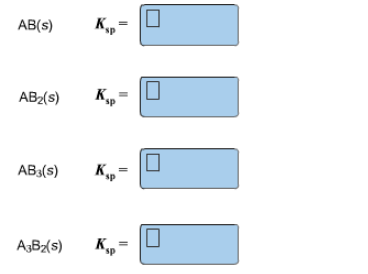# Problem: Express Ksp in terms of molar solubility, x, for a salt with a formula of

94% (22 ratings)
###### FREE Expert Solution

Each compound listed above will have a different dissociation equation.

1 - $AB_{(s)}+\rightleftharpoons A^+_{(aq)}+B^-_{(aq)}$

2 -$AB_{2(s)}\rightleftharpoons A^{2+}_{(aq)}+2\ B^-_{(aq)}$

94% (22 ratings)###### Problem Details

Express Ksp in terms of molar solubility, x, for a salt with a formula ofWhat scientific concept do you need to know in order to solve this problem?

Our tutors have indicated that to solve this problem you will need to apply the Ksp concept. You can view video lessons to learn Ksp. Or if you need more Ksp practice, you can also practice Ksp practice problems.

What is the difficulty of this problem?

Our tutors rated the difficulty ofExpress Ksp in terms of molar solubility, x, for a salt with...as medium difficulty.

How long does this problem take to solve?

Our expert Chemistry tutor, Sabrina took 7 minutes and 16 seconds to solve this problem. You can follow their steps in the video explanation above.

What professor is this problem relevant for?

Based on our data, we think this problem is relevant for Professor Hendrickson's class at UCB.